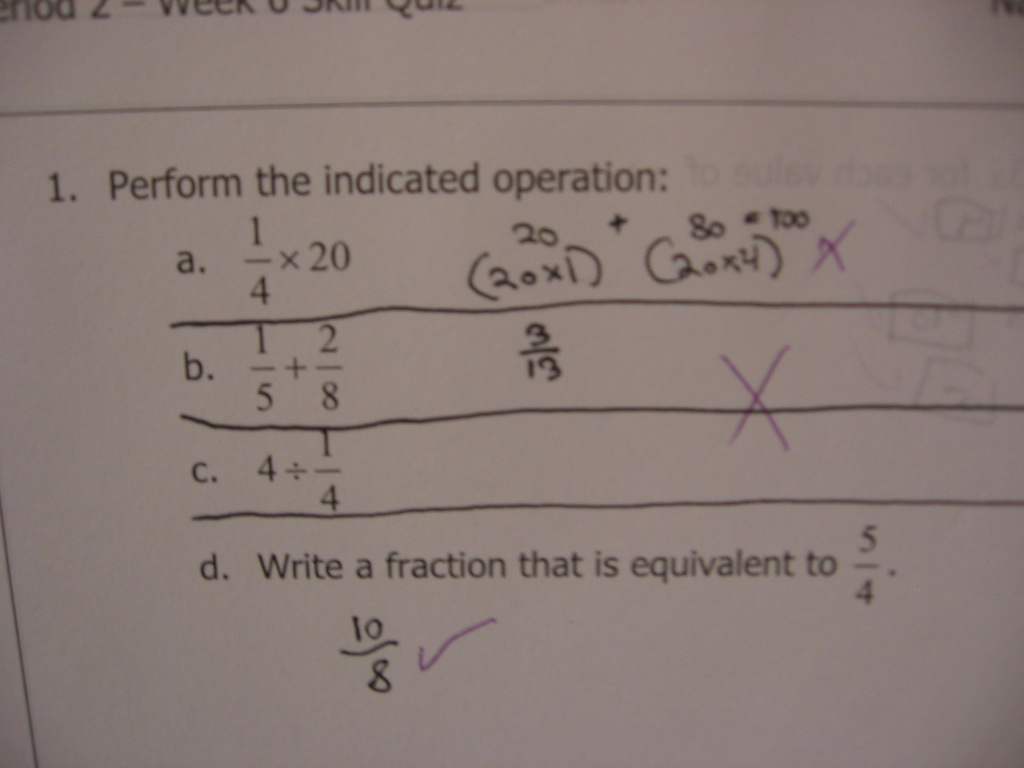Categories

# The Distributive Property of FractionsSee the title of the post.

## 6 replies on “The Distributive Property of Fractions”

Mattsays:

Letter a.). The student is using the pattern: “multiply 20 by the the numerator and denominator of the fraction”. The student does not have a conceptual understanding of both fractions and multiplying fractions. “One fourth of twenty” is going to be less than twenty and couldn’t possibly be more. So 100 should raise a red flag.

Yolandasays:

I encounter this problem a lot, students seem to think that the number “20” is equivalent to “20/20” instead of “20/1”. Seems to me, the student doesn’t really have any conceptual understanding of fractions, and is making errors with the algorithm they think they should use. Student probably knows “multiply numerators and denominators together” but then doesn’t have the math sense to realize that a fraction times a whole number has to be smaller, not bigger.

Zenosays:

Here’s my guess at an explanation:

In part b), the student used an analog of the rule for multiplication of fractions, adding the numerators to get the numerator of the result, and adding the denominators to get the denominator of the result.

In part a), the student tried to use the rule for addition of fractions, but made several mistakes. First, they incorrectly converted the integer to a fraction by using the integer as both the numerator and denominator. Then they multiplied the numerator of each fraction by the denominator of the other fraction and added to get the numerator of the result (following the rule for addition of fractions), but forgot about the denominator.

In part c), the student evidently did not have any idea how to divide an integer by a fraction. But if they had known of and attempted to apply the invert and multiply rule, they presumably would have had problems similar to those they encountered in part a).

It seems apparent that the student had not received effective instruction in the rules of arithmetic with fractions.

“It seems apparent that the student had not received effective instruction in the rules of arithmetic with fractions.”

Hahahahaha. Another fervent believer in the myth that they’ve never been taught: http://educationrealist.wordpress.com/2012/07/01/the-myth-of-they-werent-ever-taught/

I mean, spare me. The kid was taught. He was taught again and again and again. Effectively. He simply doesn’t have the cognitive skills to remember what he was taught.

There is no significance to the mistake, Michael. He just doesn’t see the effort of remembering fraction operations as worth it, so he vaguely waves a dead chicken over it.

The trick is to get the kids to stop and think with one big neon sign. So I tell my kids constantly is that they will NEVER add denominators. They will either convert and add numerators, or multiply. But never, ever add. This has some staying staying power, and has led to kids stopping “Oh, wait. I can’t add them. So which one is it? Am I going to multiply or convert?” and that much of a noodge allows them to access their memory and make a pass at actually working the problem.

mpershansays:

*nudge

-Editor

Brian Barrientossays:

If u have 6÷2(1+2) would you use the distributive property… And if u do would u use 6/2 as a fraction to distribute into 1 and 2 ?